# Constant-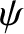Limit

Let us suppose that there are two layers in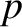space. In the small-layer, suppose that Equation (5.78) reduces to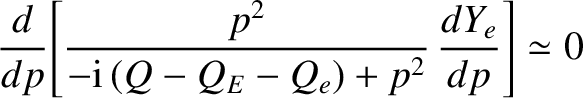(5.84)

when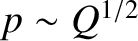. Integrating directly, we find that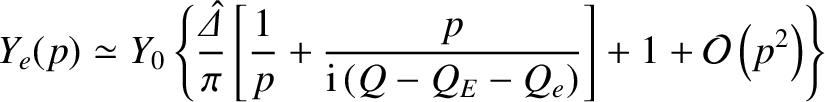(5.85)

for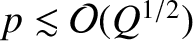, where use has been made of Equation (5.83). The two-layer approximation is equivalent to the well-known constant-approximation .

In the large-layer, for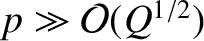, we obtain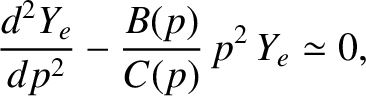(5.86)

with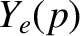bounded as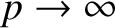. Asymptotic matching to the small-layer solution (5.85) yields the boundary condition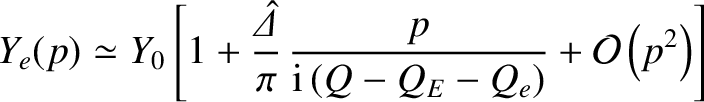(5.87)

as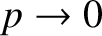.

In the various constant-linear response regimes considered in Section 5.9, Equation (5.86) reduces to an equation of the form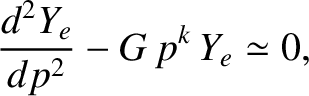(5.88)

where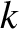is real and non-negative, and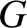is a complex constant. Let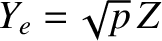and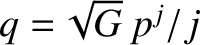, where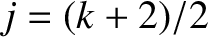. The previous equation transforms into a modified Bessel equation of general order,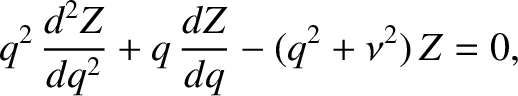(5.89)

where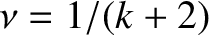. The solution that is bounded as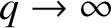has the small-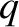expansion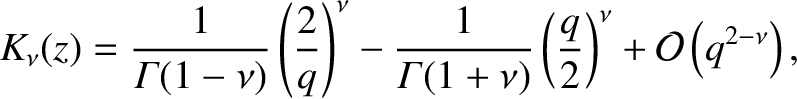(5.90)

where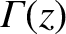is a gamma function. A comparison of this expression with Equation (5.87) reveals that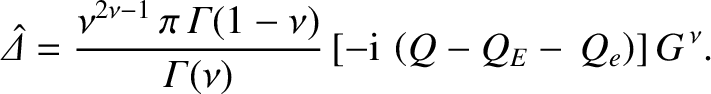(5.91)

Note, finally, that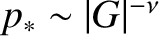, where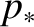denotes the width of the large-layer inspace. This width must be larger than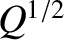(i.e., the width of the small-layer) in order for the constant-approximation to hold. Finally, it is easily demonstrated that the neglect of the term involving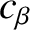in Equation (5.74) is justified provided that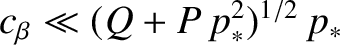.